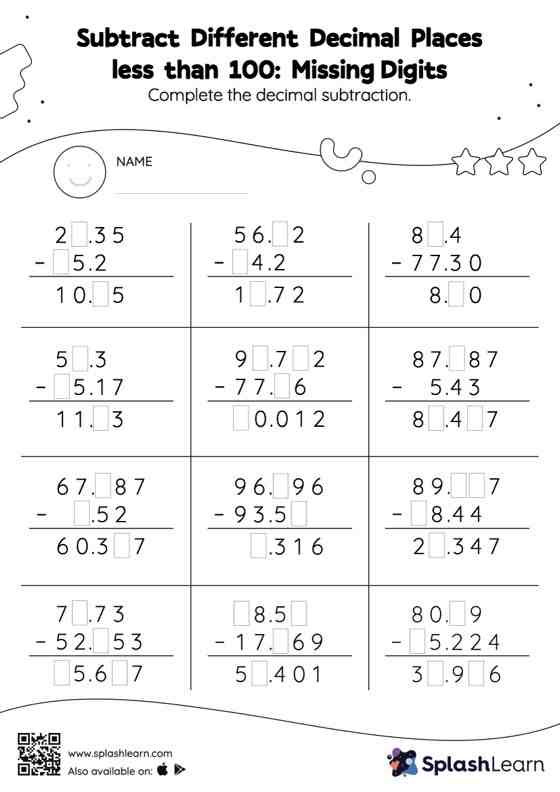# Subtract Different Decimal Places less than 100: Missing Digits Worksheet

Home > Subtract Different Decimal Places less than 100: Missing DigitsStudents align the decimal points and use zero as space holders to subtract decimals. Then they use the relationship between addition and subtraction to find the missing number in subtract different decimal places less than 100 worksheet. In this worksheet, students practice solving problems using the column method. This method is especially helpful with problems involving bigger multi-digit numbers as the format provides an easy structure to follow.